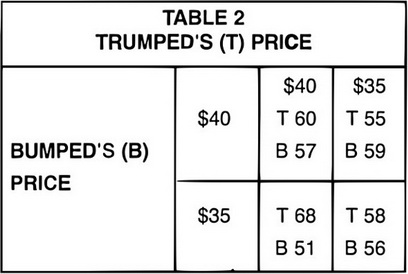# AP Microeconomics Practice Test 10

### Test Information12 questions14 minutes

1. Which of the following is an example of an economic rent?

2. The Lorenz Curve is used to measure which of the following:

3.The matrix above represents profits for two oligopolists, Trumped (T) and Bumped (B), under different pricing strategies. Which of the following is correct?

4. Which of the following statements is correct?

5. Which of the following is true for a firm operating under perfect competition in the long run?

6. Which of the following conditions is characteristic of oligopoly?

7. With respect to the kinked demand curve, which of the following is NOT correct?

8. Usually, the supply curve of firms operating under conditions of perfect competition in product market would be identified as

9. Relatively free or easy entry (low or nonexistent barriers to entry) is best matched by which of the following?

10. Which of the following represents a measure of a firm's monopoly pricing or market power, i.e., the larger the value of the index, the greater the firm's market pricing power?

11. Which of the following correctly illustrates why price (P) equals marginal revenue (MR) under perfect competition, and why price (P) is greater than marginal revenue under monopoly or imperfect competition?

I. MR = P = demand on horizontal function for perfect competition.

II. P > MR as downward sloping functions for imperfect competition.

III. Demand and Price are represented as a vertical function for imperfect competition.

12. In the design of a competitive market system, which of the following does NOT describe how resources are allocated?